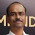# Doubt in Estimate At Completion (EAC) Calculations

Today, I got a question from our friend, PMP aspirant. He wanted me to explain the answer to the PMP question. He said that his answer is not matching the right answer given. The question is given below:
You are the project manager of a \$5 billion dollar construction project. Your project is doing extremely well in terms of cost performance, which is indicated by the Cost Performance Index value of 1.25. But, you have reasons to believe that the current variances were due to extraneous factors, and you do not expect similar variances to occur in future. The original estimated budget at completion is \$250,000. What should be the estimate at completion (EAC) for your project if AC = \$ 100,000 and EV = \$ 125,000?
OK, before going into the question, I would like to explain the various ways of calculating the Estimate At Completion (EAC). There are three different scenarios you will encounter in the EAC calculations. They are:

1. Current project performance is typical; you expect the project to perform similarly till end of the project
EAC = BAC/ CPI
Let me give you an example. Say, your original budget is \$1000. The actual cost as of now is \$200, but the earned value is only \$100. It means that you are spending \$2 for every \$1 of work. So, if the same performance continues till end of the project, you would be spending \$2000 instead of \$1000.

In terms of calculations, CPI = EV/ AC = 100/200 = 0.5
So, your EAC = BAC/ CPI = 1000/0.5 = \$2000

2. Current project performance is atypical; you expect the project to perform to original expectation from now on.
EAC = AC + (BAC-EV)
Let us use the above example in case 1. You have spent \$200 so far for an earned value of \$100. So, you still have \$900 (BAC-EV = \$1000-\$100) worth of work to be completed. Since you are expected to fall back in line with the original estimate, you need only \$900 to complete the project from now on. Add the amount you have already spent on the project (\$200).

So, EAC = 200 + (1000-100) = \$1100

3. You need to complete the project in time, irrespective of what has happened so far.
EAC= AC + (BAC-EV) / (SPI * CPI)
Let us again use the example from case 1. You have spent \$200 so far for an earned value of \$100.  But, as per schedule, you should have completed \$400 value of work.

So, PV = \$400, EV = \$100, AC = \$200
SPI = EV/PV = 100/400 = 0.25
CPI = EV/AC = 100/200 = 0.50

EAC = 200 + (1000-100)/(0.25*0.75) = \$5000.

EAC = 200 + (1000-100)/(0.25*0.50) = \$7400.

The revised estimate now is too high compared to the original estimate; this is because of the very slow progress (SPI =0.25). You may have to spend more money (using schedule compression techniques) to bring back the project on schedule.

You may also be interested in:

### Solution to the given problem

I hope you understand the different scenarios given here. Now, let me come back to the question sent by our friend, PMP Aspirant. The project manager believes that the current variances are not typical and does not expect similar variances to occur in the future. The scenario given falls under case 2. So, let us the relevant formula to compute the EAC value.

EAC = AC + (BAC-EV)
In the given problem, AC = \$100,000; BAC = \$250,000; EV = \$125,000

EAC = 100000 + (250000-125000) = \$225000

Is that clear? Do you still have any doubts? Please feel free to share your thoughts. If you need help on any other problem, I would be glad to help.

1.Anonymous

Manik,
Thank you very much for the post. Very well explained. Your explanation cleared my doubts in EAC calculations.

Please check the lines in scenario 3.

So, PV = \$400, EV = \$100, AC = \$200
SPI = EV/PV = 100/400 = 0.25
CPI = EV/AC = 100/200 = 0.50

EAC = 200 + (1000-100)/(0.25*0.75) = \$5000.

Your calculated CPI is 0.50 and in the next line applied CPI is 0.75.
It should have been
EAC = 200 + (1000-100)/(0.25*0.50) = \$8800.

Thanks!

1.Thanks for highlighting the error. I have corrected and updated the post. But, in your calculation, there is a mistake too. I have shown the steps below.

EAC= AC + {(BAC-EV) / (SPI * CPI)}
= 200 + {(1000-100)/(0.25*0.5)}
= 200 + {900/0.125}
= 200 + 7200
= \$7400

2.Anonymous

Dear,

how you got a \$400 for the PV

I dont see any number in the question says that we are on the 4th day or we are planing to spent 400 ?

1.There are two parts in this question.

First part is the main question; the answer to this question is addressed under the section "Solution to the given problem".

The second part is the explanation given in item nos. 1, 2 & 3. Here, I have explained the various scenarios you may come across. For this, I have taken arbitrarily some values just to explain the concept. So, the PV of \$400 is just an arbitrary value chosen just to explain the concept. It has nothing to do with the original question.